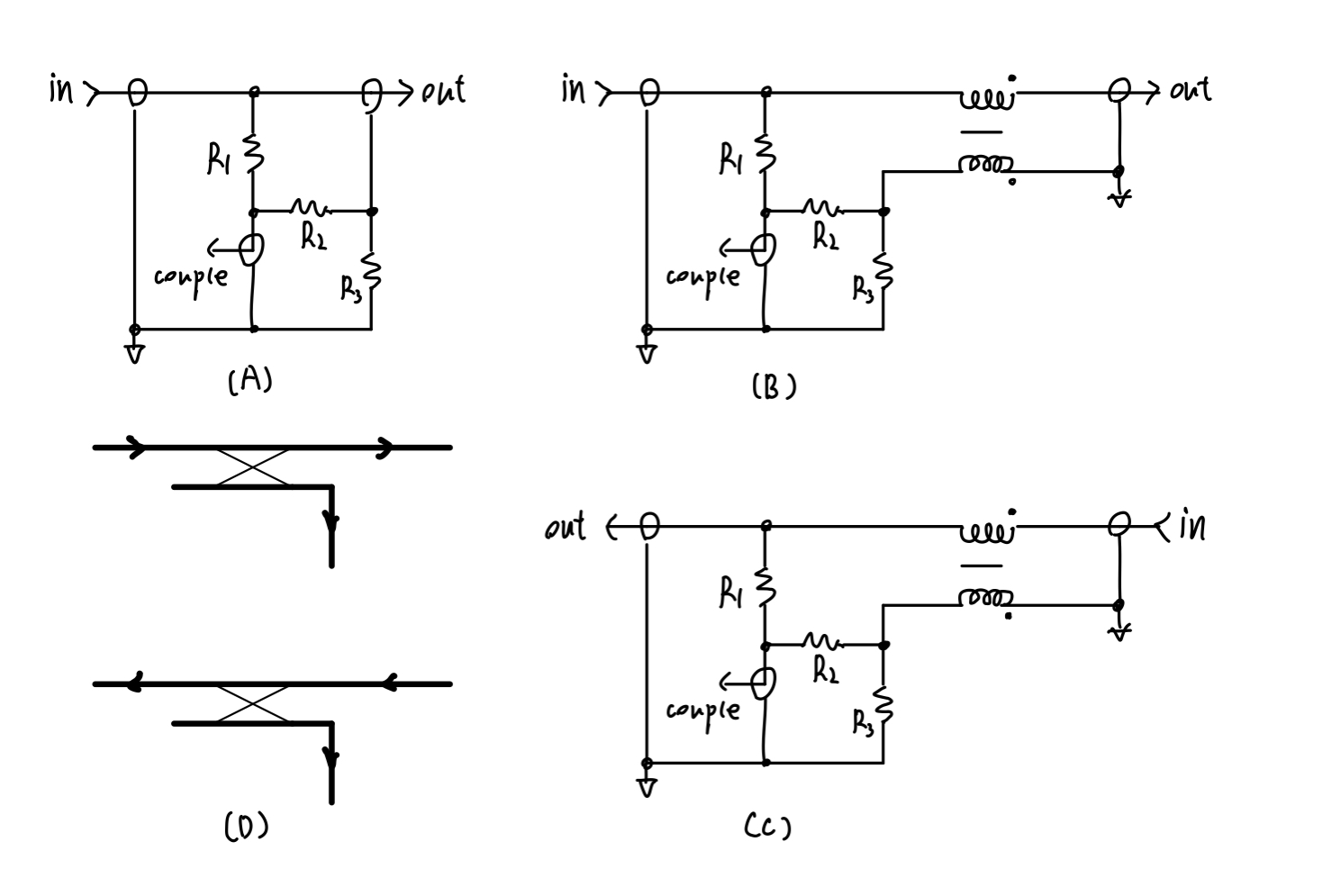# 橙的核电站

0%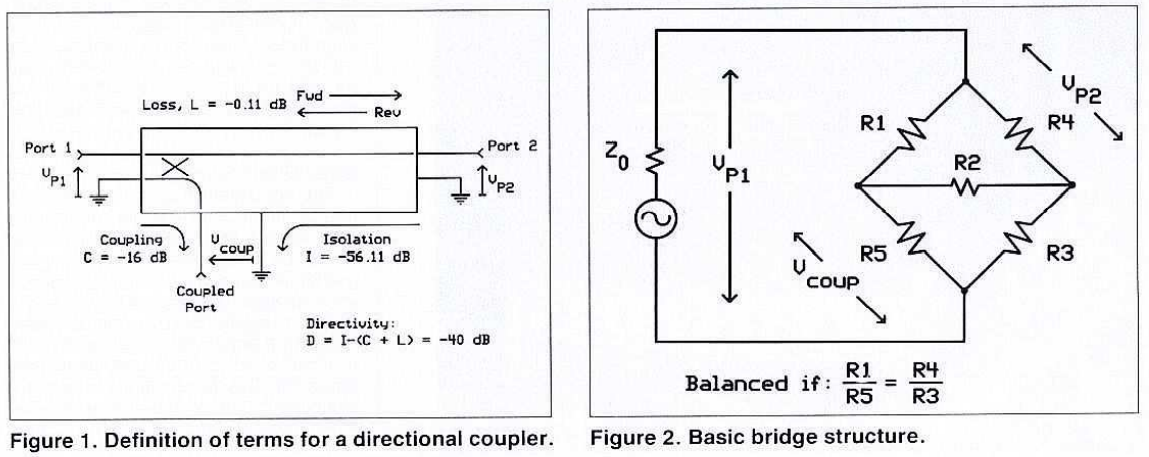## 0.引子

https://ieeexplore.ieee.org/stamp/stamp.jsp?tp=&arnumber=7345756

## 1.定向耦合器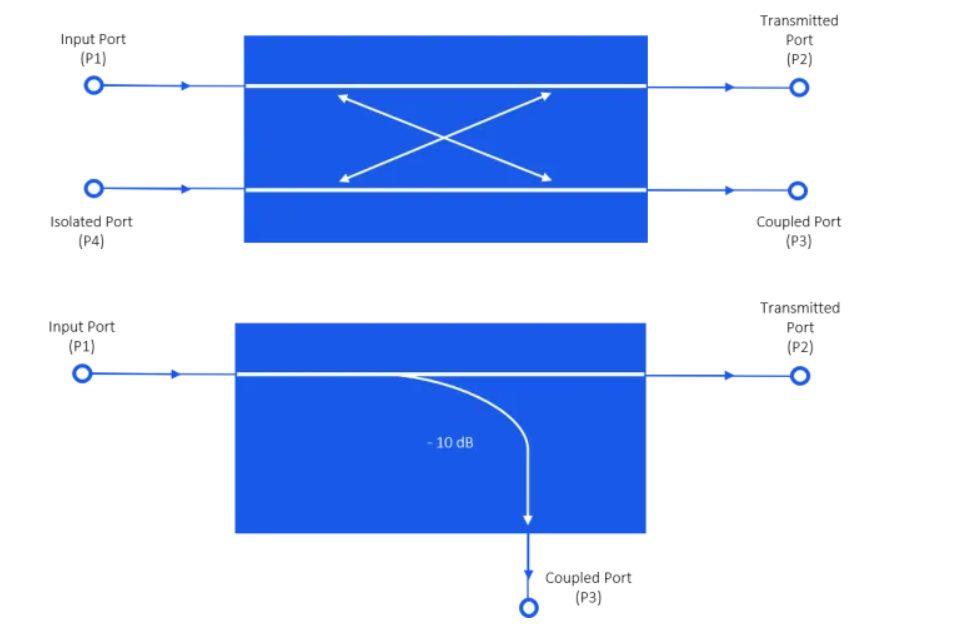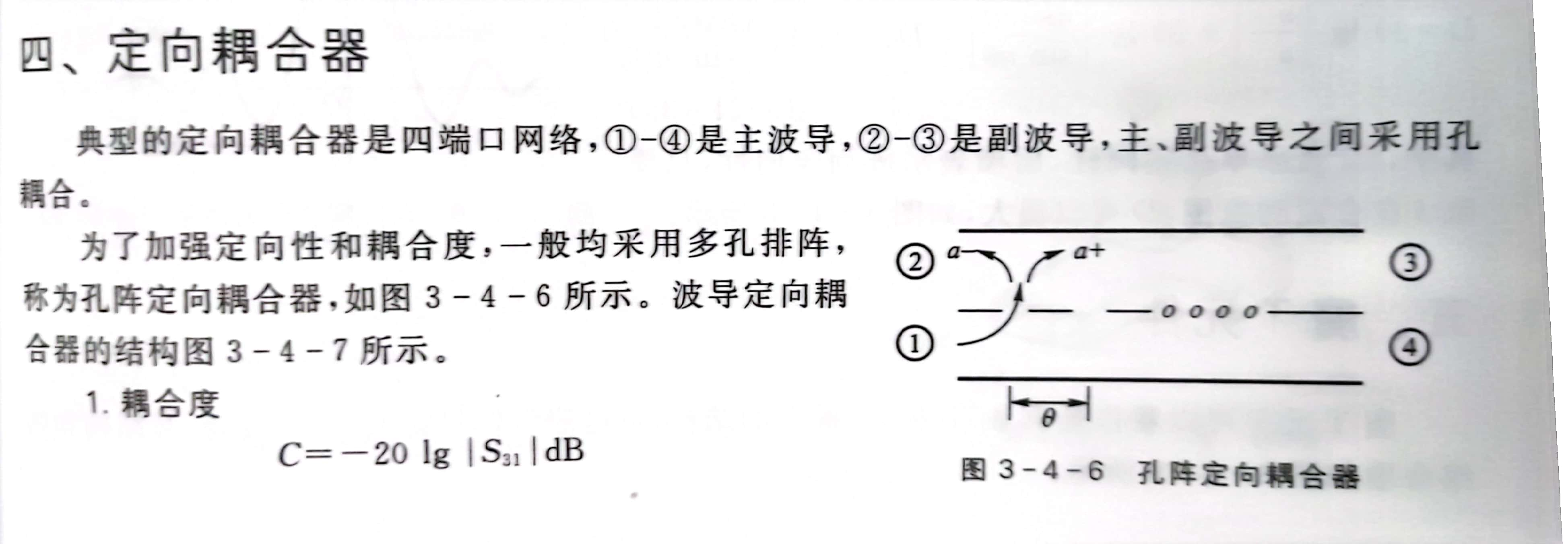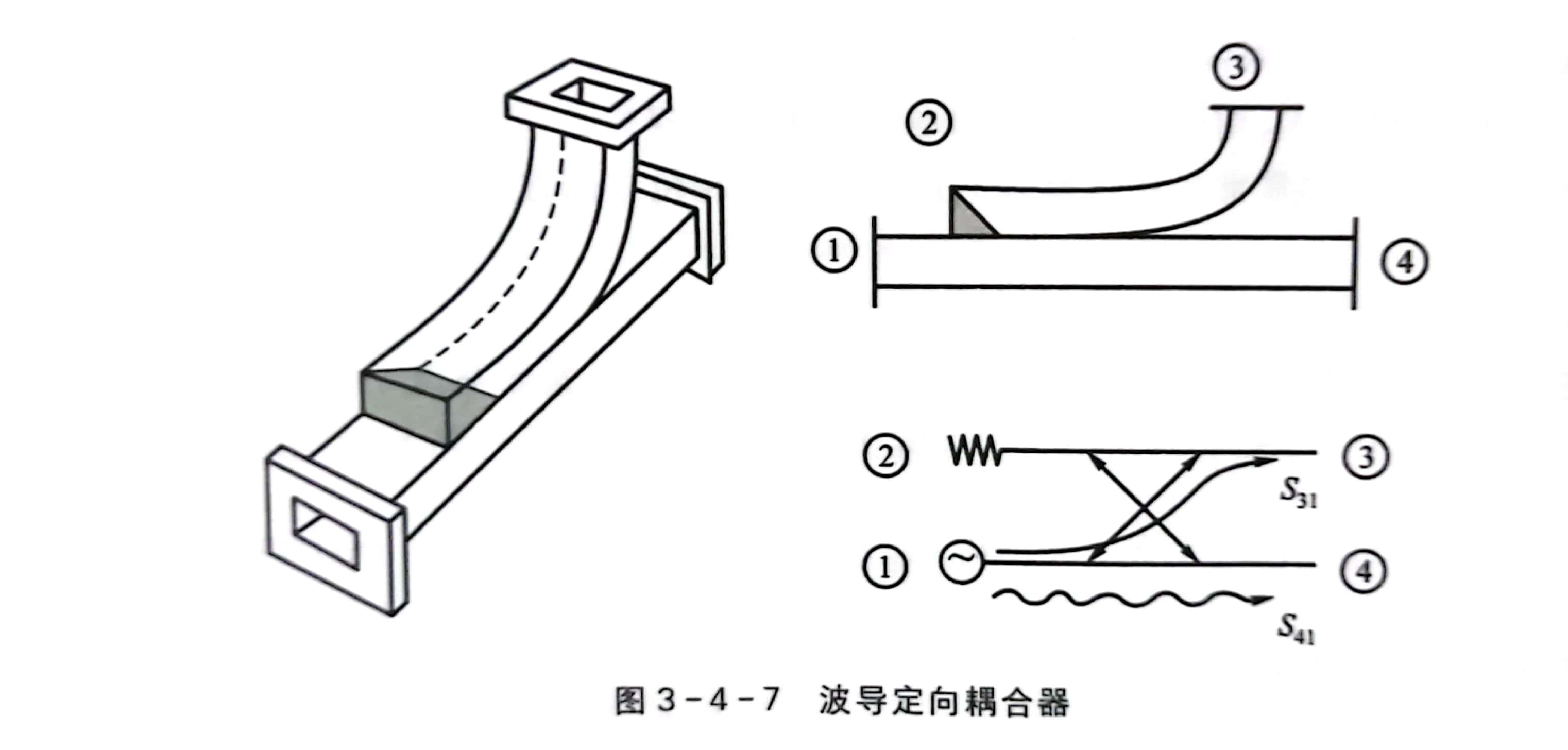## 2.定向电桥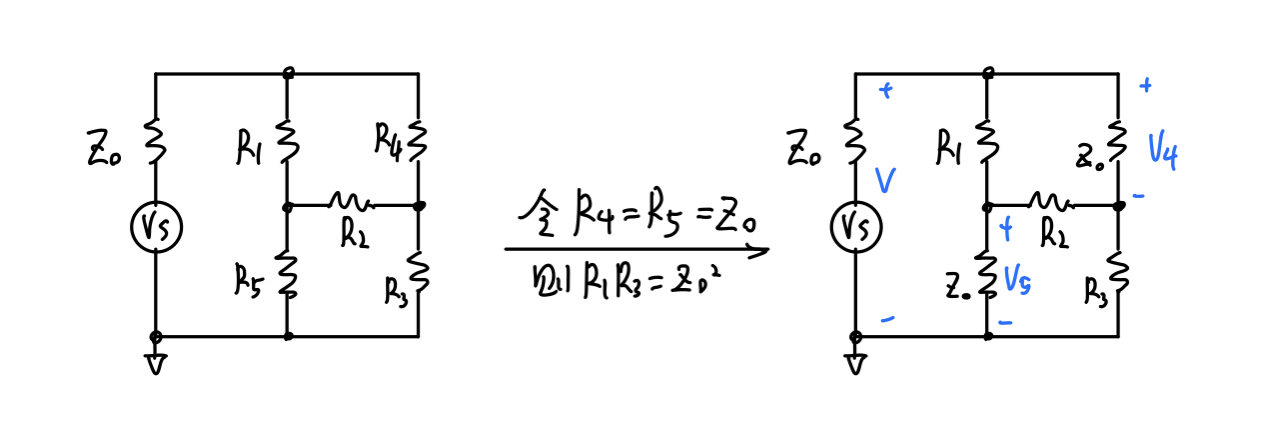$\frac{R_1}{R_5}=\frac{R_4}{R_3}，即R_1R_3=R_4R_5$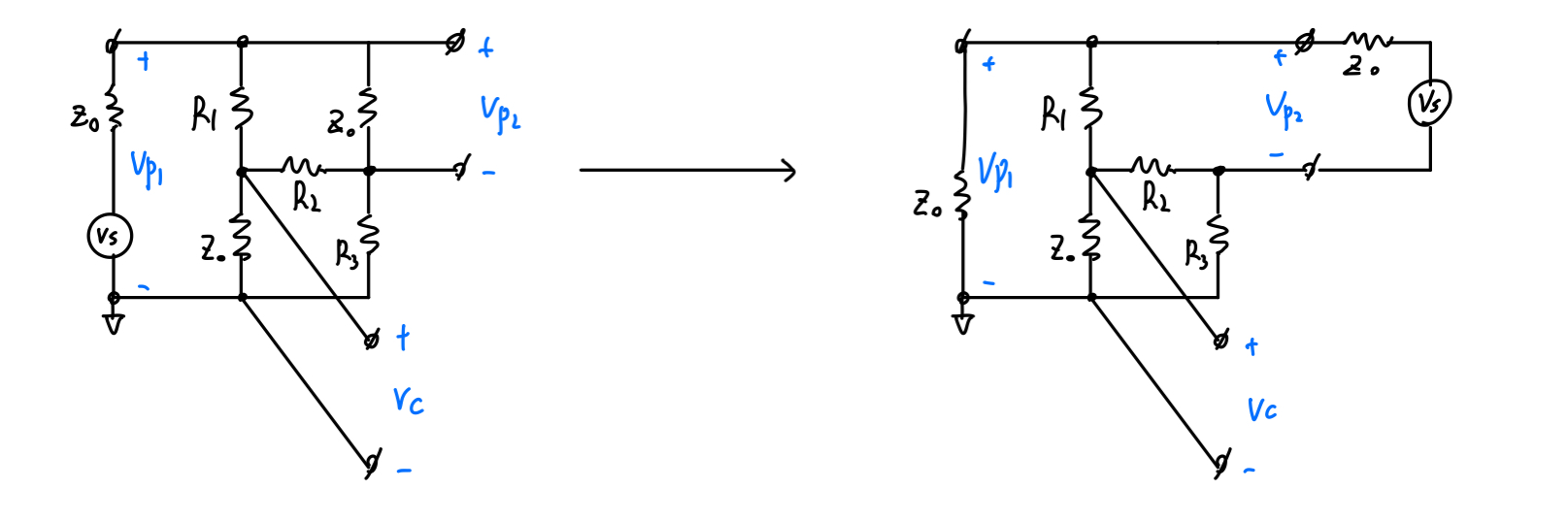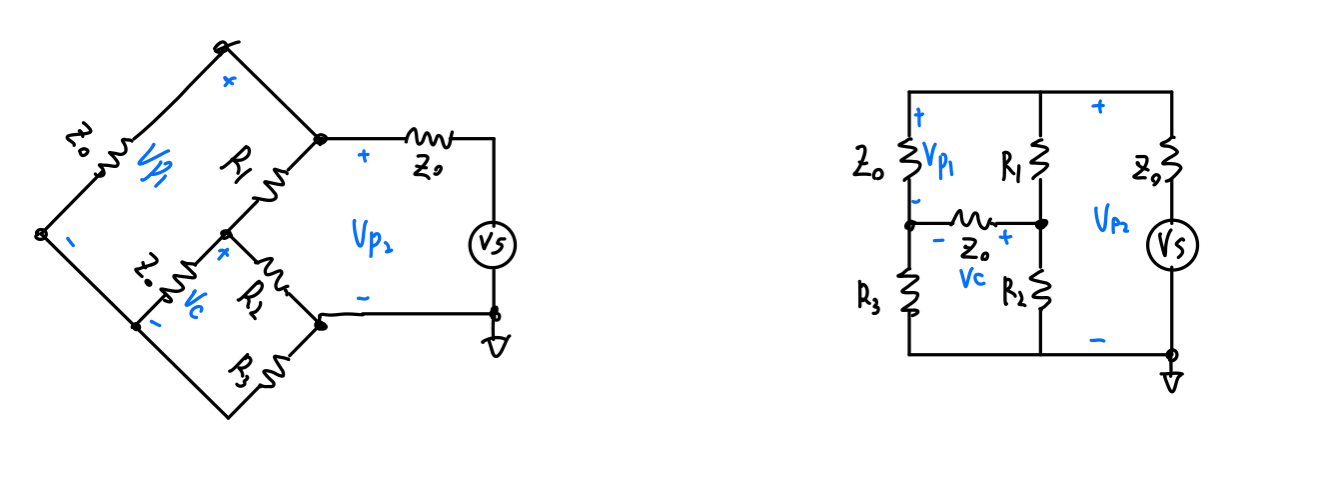$\frac{Z_0}{R_3}=\frac{R_1}{R_2}$